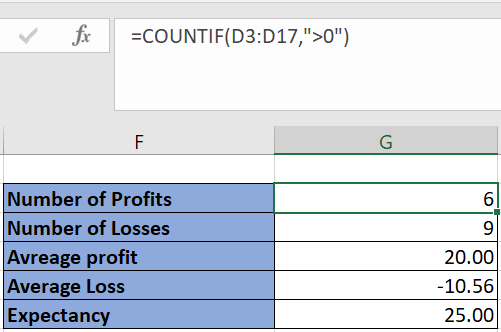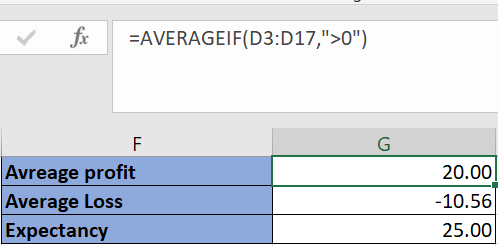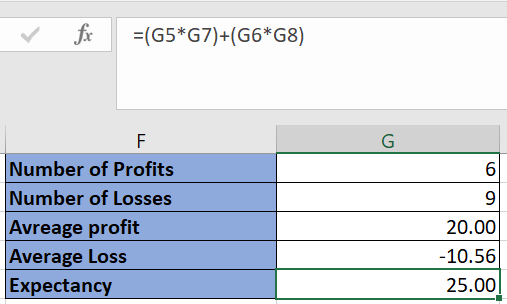Grasping the concept of Expectancy is essential for traders, as it serves as a key indicator, forecasting the potential profit or loss of each trading move. This vital metric can vary, presenting either as a positive or negative value. A positive expectancy is a clear sign of a thriving and effective trading approach. In this detailed guide, we delve into the methods to calculate expectancy with precision using Excel, providing traders with an invaluable tool for enhancing their trading strategies.

Further Reading: Methods to Compute Stop Loss in Excel

### Formula for Expectancy

Here is the formula to calculate expectancy:

Expectancy = (Total Wins * Average Win) + (Total Losses * Average Loss)

To get a reliable expectancy figure, having data for at least 15 trades is advisable.

This formula shows that a high number of wins or a high average win value leads to a positive expectancy.

It’s challenging to make every trade profitable, thus aiming for a higher average win is key, similar to focusing on the risk-reward ratio.

Modern trading analysis tools typically include expectancy in their backtesting reports.

### Implementing Expectancy Calculation in Excel

The first step in calculating expectancy is maintaining a trade log or backtest log, which records the profit and loss (P&L) of all trades.

Refer to the following image for an example:Employ the “COUNTIF” function on the P&L column for this. Use “>0” as the second parameter for counting profits and “<0” for losses.To calculate average profit and loss, use the “AVERAGEIF” function on the same column.Finally, add together the products of the number of profits and average profit and the number of losses and average loss to find expectancy.In the example above, the positive expectancy indicates an average profit of 25 per trade.

### Excel Sheet for Expectancy Calculation

Expectancy Calculation Excel Sheet### Mastering Amibroker Keyboard ShortcutsdexterNovember 24, 2023### How to Create a Strategy in Tradetron?dexterSeptember 20, 2023dexterSeptember 22, 2023